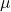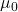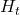# Multivariate Volatility Forecast Evaluation

The evaluation of volatility models is gracefully complicated by the fact that, unlike other time series, even the realization is not observable. Two researchers would never disagree about what was yesterday’s stock price, but they can easily disagree about what was yesterday’s stock volatility. Because we don’t observe volatility directly, each of us uses own proxy of choice. There are many ways to skin this cat (more on volatility proxy here).

In a previous post Univariate volatility forecast evaluation we considered common ways in which we can evaluate how good is our volatility model, dealing with one time-series at a time. But how do we evaluate, or compare two models in a multivariate settings, with two covariance matrices?

The solution can be found in Robert Engle’s research factory. Specifically, Testing and valuing dynamic correlations for asset allocation, Journal of Business & Economic Statistics, 2006, Vol. 24(2). The paper has around 200 citation, highly under-cited (and under-utilized if you believe the connection citations-usage).

You are welcome to read the full paper, but the main idea can be summarized quite quickly and simply. This is the key message directly from the paper:

Theorem 1 says that the conditional variance of every optimized portfolio will be greater than or equal to the conditional variance of the portfolio optimized on the true covariance matrix.

Theorem 1 offers a strategy for comparing covariance matrices, based on the idea of choosing covariance matrices that achieve lowest portfolio variance for all relevant expected returns.

The idea is quite intuitive, but it is useful that someone also formally stapled the theory behind it. So, given two estimates of covariance matrices, construct a portfolio. Out of the two covariance estimates, the portfolio would have lower variance for the more accurate covariance estimate. This will be true for any vector of expected returns and any required excess return. With this move from covariance matrix to a portfolio, you are moving from multivariate settings back to univariate settings!

Formally, ifis the vector of expected excess returns, andis the required return, you can find the portfolio weightsusingas your covariance at time:

(1)Once you have the weights, you can construct the portfolio and voila. The lower the variance of the portfolio compared to the variance of another portfolio, the better your covariance estimate. Note: the only change should be the covariance matrix, so that you compare apples to apples (no change in the vector of excess or required returns for example).

In the same paper, they show that following this idea, you can simply test the equality between two models pretty much the way it is done in the univariate case. The Diebold Mariano-type test and the Mincer Zarnowitz regression test are mentioned. I see no reason why the Jarque Bera test would not qualify here as well (but it is not mentioned in the paper).

I have shown how to estimate the covariance matrix using quite a few techniques in a series of 6 posts and counting. Now, I have pulled some history of the following tickers:  c('XLY','XLP','XLE','XLF','XLV','XLI','XLB','XLK','XLU'), and constructed global minimum variance portfolios using difference covariance estimates. I kept 1 year as an out-of-sample as well; so that we construct the portfolio based on say 9 years, then check the variance of the portfolio in a subsequent year. Here is the code:

Pull the data:

Estimate the volatility of the individual series (no covariance yet):

I use few methods for covariance estimation, all have been discussed in this blog in the past: Sample covariance (sample) CCC, DCC, equicorrelation where we shrink the covariance towards their average, equicorrelation where we shrink the covariances towards the average of the whole matrix. I also include portfolio based on simple combination of the all the covariance estimates and an equal weights portfolio.

For finding the portfolio weights I used the globalMin.portfolio (Eric Zivot and Tim Hesterberg).

Covariance estimation and portfolio construction:

Here are the results:In red, for the full sample, in black, only for the out of sample period (so 52 weeks since we use weekly data here). On the Y axis we have the annualized standard deviation of the portfolio.
We see that generally speaking, the most recent 52 weeks are less volatile than the whole sample period. A portfolio based on simple average of all five covariance estimates achieves the lowest volatility over the full sample, and is second best over the out-of-sample period. Most strikingly, the sample covariance matrix (computed based on expanding window in this case) is good enough. What I think is that since we only use 10 names, and we have quite a bit of data, the sample covariance estimate (which is also the Maximum Gaussian Likelihood) is adequate. Thinking about the other models, the DCC is motivated mainly by stylized facts (Heteroscedasticity and volatility clustering), and it is nothing more than a functional form. There is no theoretical reason for the CCC or DCC to perform better. For example, as far as I know there is no proof that shows the DCC actually converges to the real covariance matrix. As for the equicorrelation covariance, I strongly believe in the idea. Clements and friends (2015) find that shrinking is particularly helpful in times of crisis, but if the period is calm, there is not much advantage.

Main takeaway: if you have good ratio of data points to names (or number of rows to number of columns ratio), then you can argue that the sample covariance matrix, the most straight forward thing you can do, is adequate enough.

### 2 comments on “Multivariate Volatility Forecast Evaluation”

1.MANDAR PRIYA PHATAK says:

Sir,
there was an error regards to the term ” mean_rho”, wished to know how it has been defined.

1.Eran Raviv says:

It was replaced by cross_mean, so cross_mean not relevant for this post. I fixed that line.